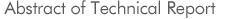No. 1317
Advanced numerical techniques in practical electromagnetic field computationInvestigating R&D Committee on advanced numerical techniques in practical electromagnetic field computation
 Keyword : Electromagnetic field analysis, high-speed and highly accurate analysis, hysteretic magnetic field analysis, iterative solution method
Numerical techniques of electromagnetic field analysis have been greatly improved in recent years with the evolution of computing machines. Numerical techniques for high-speed and highly accurate analysis have been intensely developed so as to consider the conventionally ignored phenomena leading to expanding of the scopes of demanded analysis objects. Therefore, the analysis objects become more large-scale and complicated, and the analysis techniques are required to be enhanced in a high level. A large number of useful techniques are reported for the future technical development of electromagnetic field numerical analysis through the three-year investigation since 2010. In Chapter 1, the present states and problems of various fields of electromagnetic field analysis are described, and Chapter 2 covers a various of topics, e.g., high-speed analysis techniques using infinite edge elements, fast analysis method of time-periodic steady field, the practical implementation of ICCG method, and vector hysteretic analysis methods. In Chapter 3 are described the survey of issues remained in practical electromagnetic field computation, while in Chapter 4 useful key issues and pitfall of electromagnetic field analysis.
This report will be useful for many researchers and engineers concerned with electromagnetic field analysis.

©2007. The Institute of Electrical Engineers of Japan